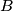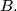### IMO Shortlist 1988 problem 21

Kvaliteta:
Avg: 0,0
Težina:
Avg: 0,0
Forty-nine students solve a set of 3 problems. The score for each problem is a whole number of points from 0 to 7. Prove that there exist two students$A$ and$B$ such that, for each problem,$A$ will score at least as many points as$B.$
Izvor: Međunarodna matematička olimpijada, shortlist 1988